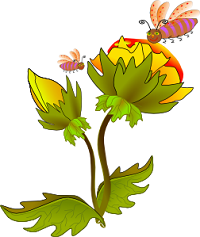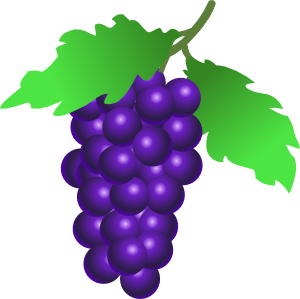# Botany Wordsearch var pfHeaderImgUrl = '';var pfHeaderTagline = '';var pfdisableClickToDel = 0;var pfHideImages = 0;var pfImageDisplayStyle = 'block';var pfDisablePDF = 0;var pfDisableEmail = 0;var pfDisablePrint = 0;var pfCustomCSS = '';var pfEncodeImages = 0;var pfShowHiddenContent = 0;var pfBtVersion='2';(function(){var js,pf;pf=document.createElement('script');pf.type='text/javascript';pf.src='//cdn.printfriendly.com/printfriendly.js';document.getElementsByTagName('head').appendChild(pf)})();M W U S Q J K M D K D L R T P Y V E Q W
R Q I N O I T A R I P S N A R T C Y J T
V Q W O O D Y O M E O L H P W D K Q P V
B Z F N D P Q O H T K E F F I V C Z H M
L C L U W H L K D I O V T T K C X T O Q
K W S T A M E N G U B L O N U M P T T R
Y O T Y B F A N Z R X O U T F L I P O X
Z E R K B K B M H F R H I O L S V I S A
H M O S G D D M E P E C R F O S R S Y D
M R C L K C I Q A B L T M S W M N T N E
T E V T M Q C T U E O T E M E A X I T I
Y P E N Q K O F H O N R J Z R C Y L H M
P S E Y H Q T M R M K I F W Y A L Q E D
M O Q L L V Y C F E J A S V T R E Y S F
A N G I O S P E R M H T C E O S M S I R
B M D Z H S E P A L Z A Y H E P B U S F
N Y G B M I G Q R E Z M M E L R K R Y N
V G N F M O I L V A I O B K C X L W D Z
C E L L L C D K O F O T B Z F A M A N S
N B B Z W J J Z H V W S T O C O N O M L1. Flowering plant ____________________________
2. Has 2 seed leaves ____________________________
3. Has 1 seed leaf ____________________________
4. "Naked seed"____________________________
5. Absorbs nutrients ____________________________
6. Openings for gas exchange ____________________________
8. Transports water ____________________________
9. Type of stem found on trees ____________________________
10. Reproductive organ of plants ____________________________
11. Insects that help pollinate____________________________
12. Photosynthetic organ ____________________________
13. Process by which plants make their food ____________________________
14. Protects the flower ____________________________
15. Main root (small roots branch from it) ____________________________
16. Waxy covering ____________________________
17. Encloses the seeds (develops from ovary) ____________________________
18. Male part of the flower ____________________________
19. Female part of the flower ____________________________
20. Evaporation from leaves ____________________________